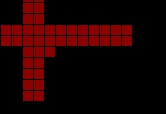# Canvas识别图片内容并使用用户头像拼接

### Logo 签到墙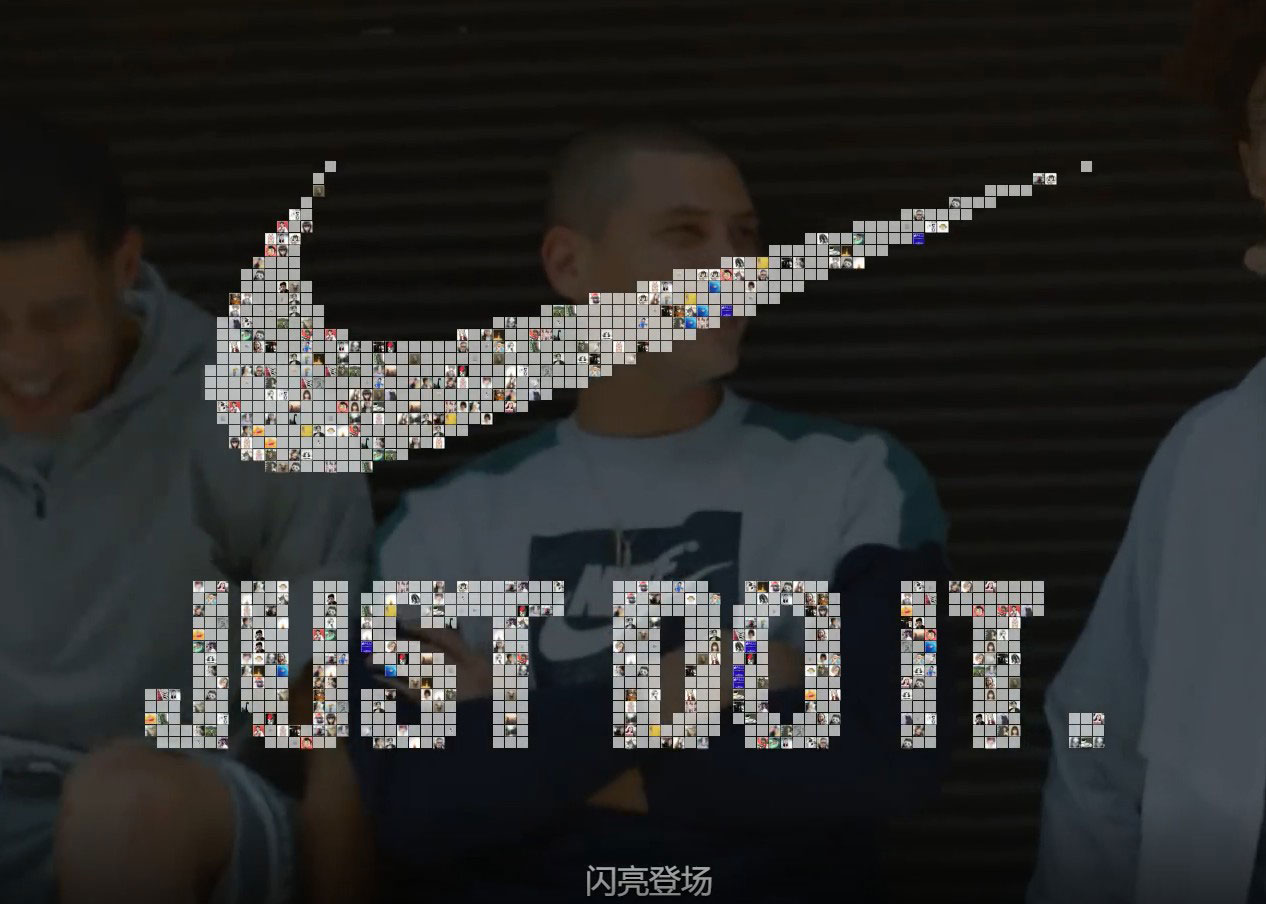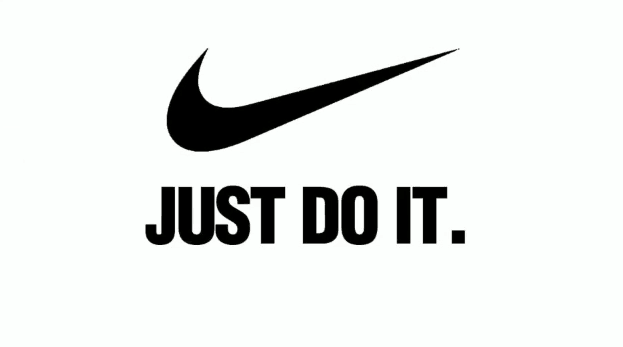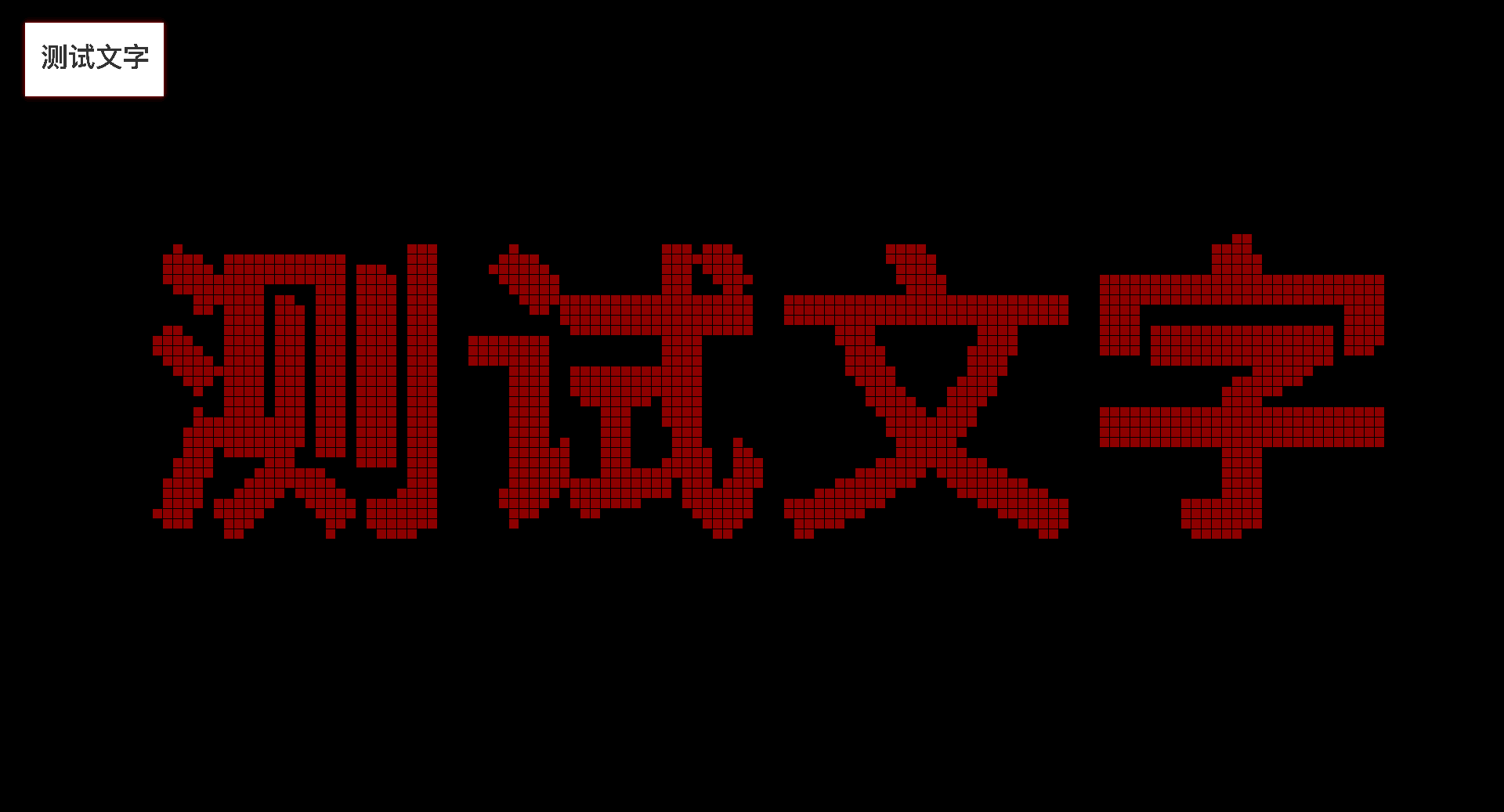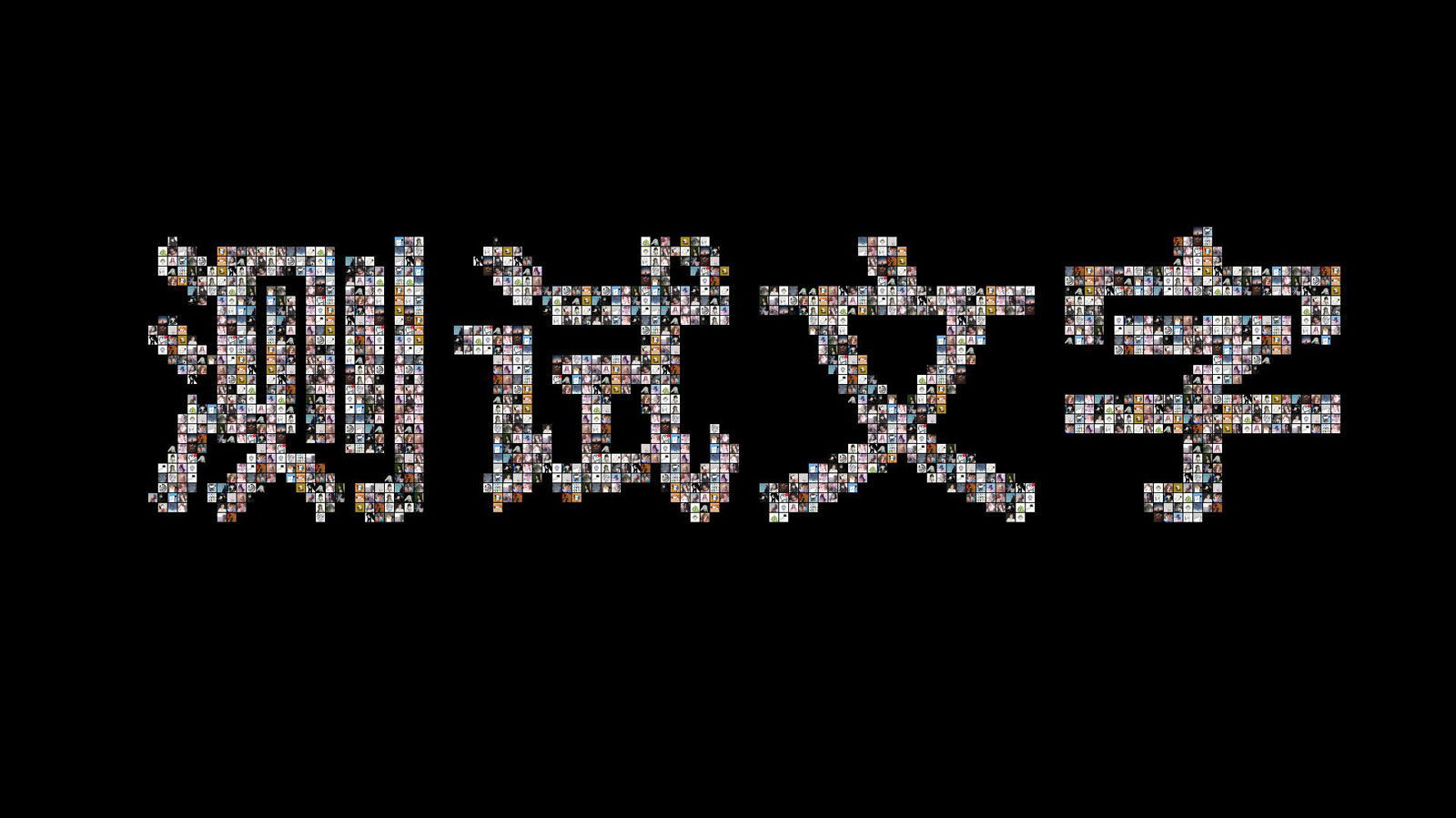#### 识别内容:

``````const maxWidth = document.body.clientWidth; // 获取视窗宽度
const maxHeight = document.body.clientHeight; // 获取视窗高度
const size = 10; // 设置栅格大小
const points = []; // 坐标数组
const canvas = document.querySelector("canvas"); // 拿到canvas实例
canvas.width = maxWidth; // 设置canvas宽度 不能使用canvas.style.width来设置，会造成内容拉伸
canvas.height = maxHeight; // 设置canvas高度
const ctx = canvas.getContext("2d"); // 获取canvas上下文，这边获取的是二维绘图，还有一个3D内容 "webgl"
let img = new Image(); // 创建图片实例
img.src = require("assets/img/text.jpg"); // 获取设置图片url
let w = maxWidth; // 设置临时宽度，后边给绘制图片的时候会用到
let h = 0; // 设置临时高度
img.onload = () => {
// img 设置 url 后会立即加载，加载完成后触发 onload 事件
// 图片加载完成
h = (w / img.width) * img.height; // 计算图片高度
ctx.drawImage(img, 0, 0, w, h); // 绘制图片从(0,0)坐标，w为绘制的图片高度，h为绘制的图片高度
// 开始识别
for (let x = 0; x <= w - 10; x += size + 1) {
// x轴开始循环 因为我需要有1像素的间隙所以是 size+1 ，如果不需要间隙则 +=size 即可
for (let y = 0; y <= h - 10; y += size + 1) {
// y轴开始循环
let color = ctx.getImageData(x, y, size, size).data; // 识别区块内容会 一个像素内返回RGBA四个参数
let count = 0; // 设置计数器
// 以4个一组开始循环
for (let i = 0; i < color.length; i += 4) {
// 如果区块中有颜色内容则 count++，我这边识别的是黑色内容
if (color[i] <= 100 || color[i + 1] <= 100 || color[i + 2] <= 100) {
count++;
}
}
// 颜色比例超过10%则记录，其实50%也可以，但是会出现 特殊情况1 的问题，下边会提到，但是内容会相对粗一些
if (count >= size * size * 0.1) {
points.push({ x: x, y: y }); // 添加到坐标数组
}
}
}
ctx.clearRect(0, 0, maxWidth, maxHeight); // 清楚绘制的图片
};``````

#### 绘制栅格图

``````ctx.fillStyle = "rgba(255,0,0,.55)"; // 设置填充色
// 遍历坐标数组内的所有数据
points.forEach(piont => {
ctx.fillRect(piont.x, piont.y, this.size, this.size); // 绘制矩形
});``````

#### 填充图片### 附

• 特殊情况 1：如果识别超过 50% 时，笔画交汇时折角会超过 50%，但是没有处在交汇处的内容可能并没有超过 50%